Latest Banking jobs   »   Quantitative Aptitude Quiz For SBI Clerk/IBPS...

# Quantitative Aptitude Quiz For SBI Clerk/IBPS RRB Clerk Mains 2021- 28th September

Directions (1 – 4): The following questions are accompanied by two statements (I) and (II). You have to determine which statements(s) is/are sufficient/necessary to answer the questions.
(a) Statement (I) alone is sufficient to answer the question but statement (II) alone is not sufficient to answer the questions.
(b) Statement (II) alone is sufficient to answer the question but statement (I) alone is not sufficient to answer the question.
(c) Both the statements taken together are necessary to answer the questions, but neither of the statements alone is sufficient to answer the question.
(d) Either statement (I) or statement (II) is sufficient to answer the question.
(e) Statements (I) and (II) together are not sufficient to answer the question.

Q1. What will volume of cylinder?
I. Height of cylinder equal to breadth of rectangle, whose perimeter is 24 cm more than perimeter of square.
II. Radius of cylinder equal to length of rectangle. Ratio between length and breadth of rectangle is 7 : 4 and side of square is half of length of rectangle.

Q2. What will be age of Veer eight years hence?
I. Four years ago ratio between age of Veer and Sameer was 6 : 7 and four years hence ratio between 1/4 th of Veer age and 1/3 rd of Sameer age will be 2 : 3.
II. Ratio between present age of Veer and Satish is 4 : 5 and that of Satish and Sameer is 7 : 8. Age of Satish is 1 years more than average age of Sameer and Veer.

Q3. ‘X’ is a two-digit positive integer. Find the value of ‘X’?
I. If digits of X are interchanged then difference between X and the interchanged number is 45.
II. If digits of X are interchanged then interchange number is 62.5% less than ‘X’.

Q4. What will be the share of R in the total profit earned by V, R and A together?
I. They together earned a profit of Rs. 54000 for a period of 1 yr.
II. R’s investment was 25% less than V’s and 50% more than A’s.

Directions (5-8): The following questions are accompanied by three statements A, B and C. You have to determine which statement(s) is/are necessary/sufficient to answer the question.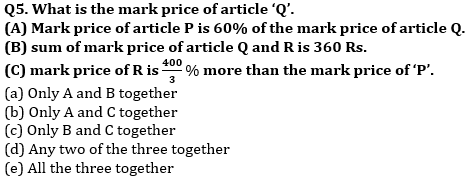Q6. Calculate the speed of stream ?
(A) To cover 32 km upstream and 32km downstream by boat, time difference is 4 hour.
(B) to cover 24 km upstream and downstream by boat, time difference is 3 hour.
(C) boat can cover 60 km in upstream in 6 hour if it double its speed.
(a) Only A and B together
(b) Only A and C together
(c) Only C and A or C and B together
(d) Any two of the three together
(e) All the three together

Q7. What is the simple interest earned in two year on principal ?
(A) The difference between compound interest and simple intrest earned in 2 year on a certain principle is Rs150.
(B) Interest earned after 3 year on principle at C.I. is 4965 Rs.
(C) Principle become equal to SI in 10 years.
(a) Only A and B together
(b) Only C and B or C and A together
(c) Only B and C together
(d) Any two of the three together
(e) All the three together

Q8. Find the number of girls.
(A) Average weight of boys is 36 kg.
(B) Total strength of class is 80 and have 33.15 kg average weight.
(C) average weight of girls is 30 kg.
(a) Only A and B together
(b) Only A and C together
(c) Only B and C together
(d) Any two of the three together
(e) All the three together

Directions (9-10): The following questions are accompanied by two statements A and B. You have to determine which statements(s) is/are sufficient/necessary to answer the questions.
(a) Statement A alone is sufficient to answer the question but statement B alone is not sufficient to answer the questions.
(b) Statement B alone is sufficient to answer the question but statement A alone is not sufficient to answer the question.
(c) Both the statements taken together are necessary to answer the questions, but neither of the statements alone is sufficient to answer the question.
(d) Either statement A or statement B by itself is sufficient to answer the question.
(e) Statements A and B taken together are not sufficient to answer the question.

Q9. Find the overall profit or loss percentage of retailer if he sells two bats and four balls.
(A) Cost price of 3 bats is equal to selling price of 4 balls while profit percentage on selling one bat is 50%.
(B) On selling two bats retailer earn 50% profit while on selling four balls retailers loss 40% of his money.

Q10. A box contains 4 green ball, ‘x’ blue balls, 5 violet balls and ‘y’ yellow balls. Find the value of ‘x + y’.
(A) Probability of choosing two green ball is 1/20.
(B) Probability of choosing one blue ball or one violet ball is ½.

Solutions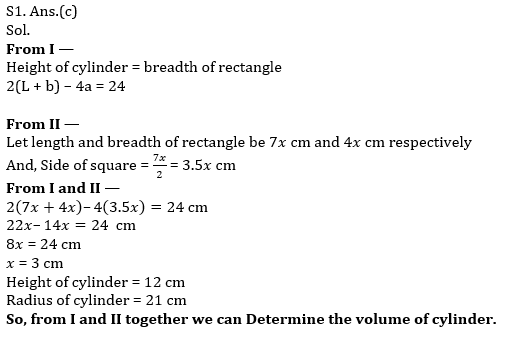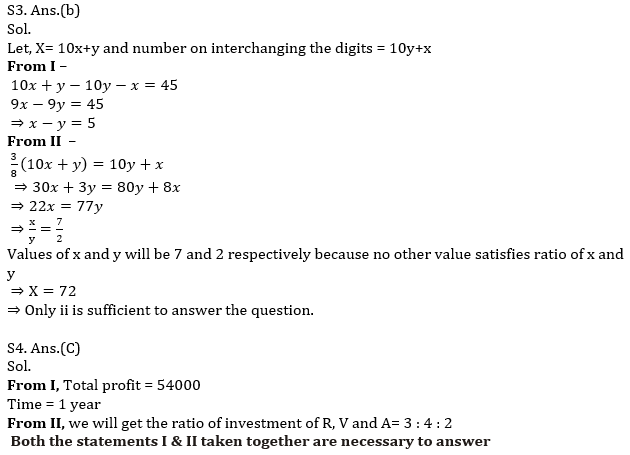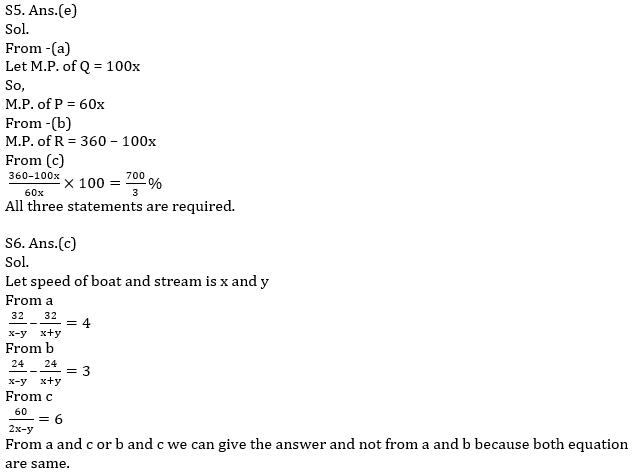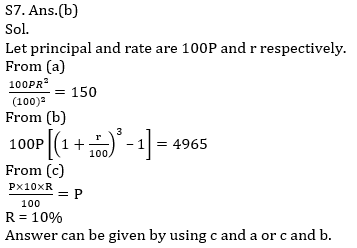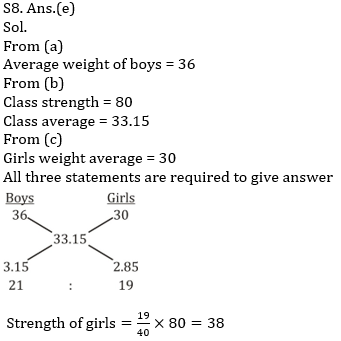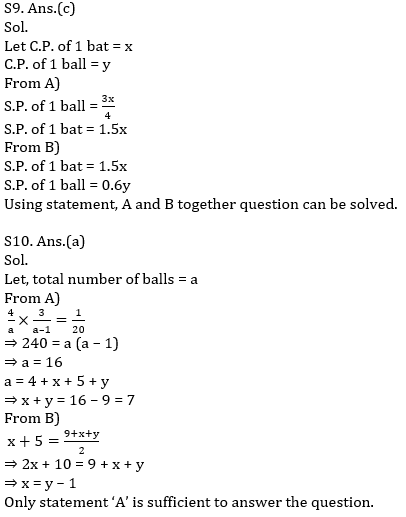Click Here to Register for Bank Exams 2021 Preparation Material#### Congratulations!Download Hindu Review of October 2021: Free PDF